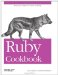# Picking a Random Line from a File

Problem

You want to choose a random line from a file, without loading the entire file into memory.

Solution

Iterate over the file, giving each line a chance to be the randomly selected one:

```	module Enumerable
def random_line
selected = nil
each_with_index { |line, lineno| selected = line if rand < 1.0/lineno }
return selected.chomp if selected
end
end

#Create a file with 1000 lines
open('random_line_test', 'w') do |f|
1000.times { |i| f.puts "Line #{i}" }
end

#Pick
random lines from the file.
f = open('random_line_test')
f.random_line # => "Line 520"
f.random_line # => nil
f.rewind
f.random_line # => "Line 727"
```

Discussion

The obvious solution reads the entire file into memory;

```	File.open('random_line_test') do |f|
l[rand(l.size)].chomp
end
# => "Line 708"
```

The recommended solution is just as fast, and only reads one line at a time into memory. However, once it's done, the file pointer has been set to the end of the file and you can't access the file anymore without calling File#rewind. If you want to pick a lot of random lines from a file, reading the entire file into memory might be preferable to iterating over it multiple times.

This recipe makes for a good command-line tool. The following code uses the special variable \$., which holds the number of the line most recently read from a file:

```	\$ ruby -e 'rand < 1.0/\$. and line = \$_ while gets; puts line.chomp if line'
```

The algorithm works because, although lines that come earlier in the file have a better chance of being selected initially, they also have more chances to be replaced by a later line. A proof by induction demonstrates that the algorithm gives equal weight to each line in the file.

The base case is a file of a single line, where it will obviously work: any value of Kernel#rand will be less than 1, so the first line will always be chosen.

Now for the inductive step. Assume that the algorithm works for a file of n lines: that is, each of the first n lines has a 1/n chance of being chosen. Then, add another line to the file and process the new line. The chance that line n+1 will become the randomly chosen line is 1/(n+1). The remaining probability, n/n+1, is the chance that one of the other n lines is the randomly chosen one.

Our inductive assumption was that each of the n original lines had an equal chance of being chosen, so this remaining n/n+1 probability must be distributed evenly across the n original lines. Given a line in the first n, what's it's chance of being the chosen one? It's just n/n+1 divided by n,or 1/n+1. Line n+1 and all earlier lines have a 1/n+1 chance of being chosen, so the choice is truly random.

• Recipe 2.5, "Generating Random Numbers"
• Recipe 4.10, "Shuffling an Array"
• Recipe 5.11, "Choosing Randomly from a Weighted List"Ruby Cookbook (Cookbooks (OReilly))
ISBN: 0596523696
EAN: 2147483647
Year: N/A
Pages: 399

Similar book on Amazon